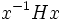# Tour:Right coset of a subgroup

PREVIOUS: Left cosets are in bijection via left multiplication| UP: Introduction three (beginners)| NEXT: Left and right coset spaces are naturally isomorphic
General instructions for the tour | Pedagogical notes for the tour | Pedagogical notes for this part
WHAT YOU NEED TO DO:
• Read the various definitions of right coset given below, and prove that they're equivalent (ignore the definition involving group action)

## Definition

### Definition with symbols

Let$H$ be a subgroup of a group$G$. Then, a right coset of$H$ is a nonempty subset$S \subset G$ satisfying the following equivalent properties:

1.$xy^{-1}$ is in$H$ for any$x$ and$y$ in$S$, and for any fixed$y$, the map$x \mapsto xy^{-1}$ is a surjection from$S$ to$H$
2. There exists a$g$ in$G$ such that$S = Hg$
3. For any$x$ in$S$,$S = Hx$
PONDER (WILL BE EXPLORED LATER): How do the definitions of left and right coset differ? Does every statement made for left cosets have a natural analogue for right cosets?
WHAT'S MORE: Some further facts about right cosets, similar to the facts we saw about left cosets. Ignore unfamiliar terminology.

## Facts

### Right congruence

The right cosets of a subgroup are pairwise disjoint, and hence form a partition of the group. The relation of being in the same right coset is an equivalence relation on the group, and this equivalence relation is termed the right congruence induced by the subgroup.

### Relation with left coset

Every subset that occurs as a right coset of a subgroup also occurs as a left coset. In fact, the right coset$Hx$ occurs as the left coset$x(x^{-1}Hx)$ with$x^{-1}Hx$ being the new subgroup.

### Condition for a left coset to also be a right coset

For a given group$H$, a subset is both a left coset of$H$ and a right coset of$H$ if it is of the form$xH$ where$x$ is in the normalizer of$H$. In other words, the normalizer of a subgroup can be defined as the union of those subsets that are both left and right cosets of$H$.

## Numerical facts

### Size of each right coset

Let$H$ be a subgroup of$G$ and$x$ be any element of$G$. Then, the map sending$g$ in$H$ to$gx$ is a bijection from$H$ to$Hx$.

### Number of right cosets

The number of right cosets of a subgroup is termed the index of that subgroup.

Since all right cosets have the same size as the subgroup, we have a formula for the index of the subgroup when the whole group is finite: it is the ratio of the order of the group to the order of the subgroup.

This incidentally also proves Lagrange's theorem -- the order of any subgroup of a finite group divides the order of the whole group.

## Natural isomorphism of left cosets with right cosets

There is a natural bijection between the set of left cosets of a subgroup and the set of right cosets of that subgroup. This bijection arises from the natural antiautomorphism of a group defined by the map sending each element to its inverse. Further information: Left and right coset spaces are naturally isomorphic